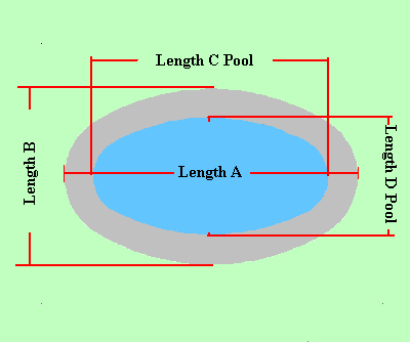Spike's Calculators

# Oval Shaped Concrete Slab - Oval Shaped Pool - Metric Measurements

Calculate the amount of concrete needed to pour a slab around an oval shaped pool using metric measurements. The slab will have the same oval shaped as the pool.

Includes the square metres of the slab around the pool. The square metres of this area can be used to estimate different materials and the perimeter of the outer area can be used to estimate the fencing material.### Oval Shaped Pool Slab - Oval Pool

Length A m
Length B m
Length C of Pool m
Length D of Pool m
Required Slab Depth mm

#### Results:

 Area of Pool m² Area of Pool Patio m² Outer Perimeter lin m Concrete Needed in Cubic Metres m³ Concrete Needed in Cubic Yards yd³

#### Calculations

1. the overall area length (length A in metres)
2. the overall area width (length B in metres)
3. length of pool (length C in metres)
4. width of pool (length D in metres)
5. depth of concrete slab (millimetres)

#### Results

1. square metres of the pool
2. square metres of the finished area around the pool
3. perimeter of outer area (lineal metres)
4. concrete needed in cubic metres
5. concrete needed in cubic yards